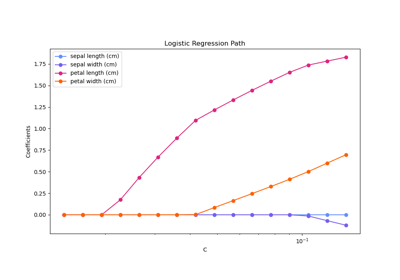# sklearn.svm.l1_min_c¶

sklearn.svm.l1_min_c(X, y, *, loss='squared_hinge', fit_intercept=True, intercept_scaling=1.0)[source]

Return the lowest bound for C.

The lower bound for C is computed such that for C in (l1_min_C, infinity) the model is guaranteed not to be empty. This applies to l1 penalized classifiers, such as LinearSVC with penalty=’l1’ and linear_model.LogisticRegression with penalty=’l1’.

This value is valid if class_weight parameter in fit() is not set.

Parameters:
X{array-like, sparse matrix} of shape (n_samples, n_features)

Training vector, where n_samples is the number of samples and n_features is the number of features.

yarray-like of shape (n_samples,)

Target vector relative to X.

loss{‘squared_hinge’, ‘log’}, default=’squared_hinge’

Specifies the loss function. With ‘squared_hinge’ it is the squared hinge loss (a.k.a. L2 loss). With ‘log’ it is the loss of logistic regression models.

fit_interceptbool, default=True

Specifies if the intercept should be fitted by the model. It must match the fit() method parameter.

intercept_scalingfloat, default=1.0

When fit_intercept is True, instance vector x becomes [x, intercept_scaling], i.e. a “synthetic” feature with constant value equals to intercept_scaling is appended to the instance vector. It must match the fit() method parameter.

Returns:
l1_min_cfloat

Minimum value for C.

## Examples using sklearn.svm.l1_min_c¶Regularization path of L1- Logistic Regression

Regularization path of L1- Logistic Regression